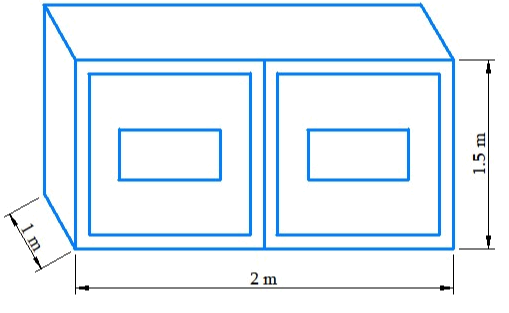# Ex.11.3 Q4 Mensuration Solution - NCERT Maths Class 8

Go back to  'Ex.11.3'

## Question

Rukhsar painted the outside of the cabinet of measure $$1\,\rm{m} \times 2\, \rm{m} \times \,1.5\, \rm{m.}$$ How much surface area did she cover if she painted all except the bottom of the cabinet?Video Solution
Mensuration
Ex 11.3 | Question 4

## Text Solution

What is Known?

Dimensions of the cuboid.

What is unknown?

Surface area of cuboid that was palindrome.

Reasoning:

Surface area of bottom of the cuboid will be subtracted from the total surface area of the cabinet.

Steps:

Length ($$l$$) of the cabinet $$=\,2\,\rm{m}$$

Breadth ($$b$$) of the cabinet $$=1\,\rm{m}$$

Height ($$n$$) of the cabinet $$= 1.5\,\rm{m}$$

Area of the bottom of cabinet $$= (l \times b)$$

Total Surface area of the cabinet $$= 2(l \times b + b \times h + n \times l)$$

Area of the cabinet that was painted $$=$$ (Total surface area of  the cabinet) $$-$$ (Area of bottom of the cabinet)

\begin{align} &= 2(l \times b + b \times h + h \times l) - l \times b\\&= 2(b \times h + h \times l) + l \times b\\ &= [2 \times (1 \times 1.5 + 1.5 \times 2) + 2 \times 1]\,{{\rm{m}}^2}\\&= [2 \times 4.5 + 2]\,{{\rm{m}}^2}\\&= 11\,{{\rm{m}}^2} \end{align}

Hence, the required area $$= 11 \,\rm{m}^2$$

Learn from the best math teachers and top your exams

• Live one on one classroom and doubt clearing
• Practice worksheets in and after class for conceptual clarity
• Personalized curriculum to keep up with school